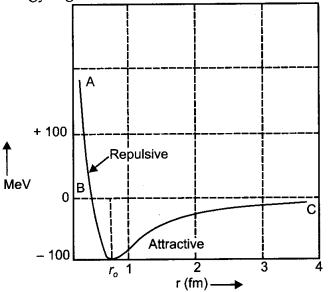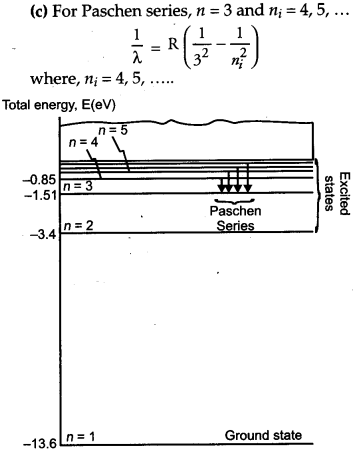## CBSE Previous Year Question Papers Class 12 Physics 2013 Delhi

### CBSE Previous Year Question Papers Class 12 Physics 2013 Delhi Set-I

Question 1.
What are permanent magnets ? Give one example. 
The magnets which have high retentivity and high coercivity are known as permanent magnets.
For example : Steel, cobalt

Question 2.
What is the geometrical shape of equipotential surface due to a single isolated charge ? 
The equipotential surfaces of an isolated charge are concentric spherical shells and the distance between the shells increase with the decrease in electric field and vice-versa.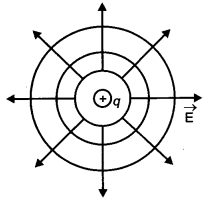Question 3.
Which of the following waves can be polarized
(i) Heat waves
Heat waves can be polarized as they are transverse waves whereas sound waves cannot be polarized as they are longitudinal waves.

Transverse waves can oscillate in the direction perpendicular to the direction of its transmission but longitudinal waves oscillate only along the direction of its transmission. So, longitudinal
waves cannot be polarized.

Question 4.
A capacitor has been charged by a dc source. What are the magnitude of conduction and displacement current, when it is fully charged ? 
Answer: Electric flux through plates of capacitor,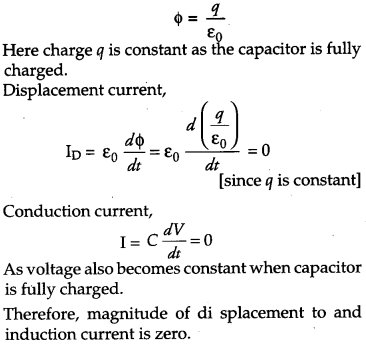Question 5.
Write the relationship between angle of incidence ‘ i ‘ angle of prism ‘A’ and angle of minimum deviations δm for a triangular prism. 
The relation between the angle of incidence i, angle of prism A, and the angle of minimum deviation δm, for a triangular prism is given by $$i=\frac{\mathrm{A}+\delta_{m}}{2}$$

Question 6.
The given graph shows the variation of photoelectric current (I) versus applied voltage (V) for two different photosensitive materials and for two different intensities of the incident radiations. Identify the pairs of curves that correspond to different materials but same intensity of incident radiation.Curves 1 and 2 correspond to similar materials while curves 3 and 4 represent different materials, since the value of stopping potential for 1, 2 and 3, 4 are the same. For the given frequency of the incident radiation, the stopping potential is independent of its intensity.
So, the pairs of curves (1 and 3) and (2 and 4) correspond to different materials but same intensity of incident radiation.

Question 7.
A 10 V battery of negligible internal resistance is connected across a 200 V battery and a resistance of 38 Ω as shown in the figure. Find the value of the current in circuit.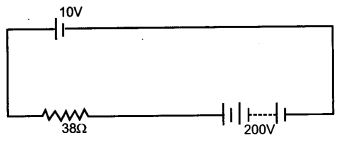Since, the positive terminal of the batteries are connected together (So they oppose each other), so the equivalent emf of the batteries is given by
E = 200 – 10 = 190 V
Hence, the current in the circuit is given by
$$I=\frac{E}{R}=\frac{190}{38}=5 \mathrm{A}$$

Question 8.
The emf of a cell is always greater than its terminal voltage. Why ? Give reason. 
The emf of a cell is greater than its terminal voltage because there is some potential drop across the cell due to its small internal resistance.

Question 9.
(a) Write the necessary conditions for the phenomenon of total internal reflection to occur.
(b) Write the relation between the refractive index and critical angle for a given pair of optical media. 
(a) Necessary conditions for total internal reflection to occur are :

1. The incident ray on the interface should travel in optically denser medium.
2. The angle of incidence should be greater than the critical angle for the given pair of optical media.

(b) $$a_{\mu_{b}}=\frac{1}{\sin C}$$
Where a and b are the rarer and denser media respectively. C is the critical angle for the given pair of optical media.

Question 10.
State Lenz’s law.
A metallic rod held horizontally along east- west direction, is allowed to fall under gravity. Will there be an emf induced at its ends ? Justify your answer. 
Lenz’s law states that the polarity of induced emf is such that it produces a current which opposes the change in magnetic flux that produces it. Yes, emf will be induced in the rod as there is change in magnetic flux. When a metallic rod held horizontally along east- west direction, is allowed to fall freely under gravity i.e., fall from north to south, the magnetic flux changes due to vertical component of Earth’s magnetic field, which keeps on changing and the emf is induced in it.

Question 11.
A convex lens of focal length 25 m is placed coaxially in contact with a concave lens of focal length 20 cm. Determine the power of the combination. Will the system be converging or diverging in nature ? 
We have, focal length of convex lens,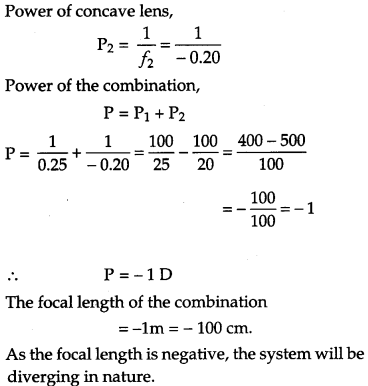Question 12.
An ammeter of resistance 0.80 Ω can measure current up to 1.0 A. 
(i) What must be the value of shunt resistance to enable the ammeter to measure current up to 5.0 A ?
(ii) What is the combined resistance of the ammeter and the shunt ?
We have, resistance of ammeter, RA = 0.80 Ω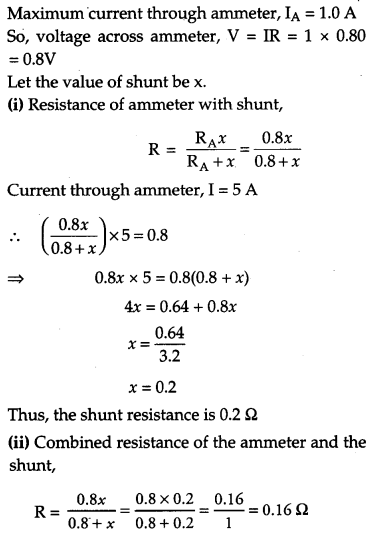Question 13.
In the given circuit diagram a voltmeter ‘V’ is connected across a lamp ‘L’. How would
(i) the brightness of the lamp and
(ii) voltmeter reading ‘V’ be affected, if the value of resistance ‘R’ is decreased ? Justify your answer.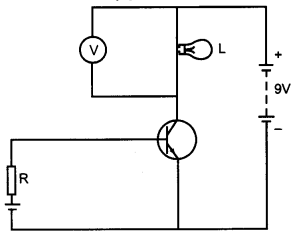The given figure is Common Emitter (CE) configuration of an n-p-n transistor. The input circuit is forward biased and collector circuit is reverse biased.

If resistance R decreases, forward biased in the input circuit will increase, thus the base current (IB) will decrease and the emitter current (IE) will increase. This will increase the collector current
(IC) as IE = IB + IC.

When IC increases which flows through the lamp, the voltage across the bulb will also increase making the lamp brighter and since the voltmeter is connected in parallel with the lamp, . the reading in the voltmeter will also increase.

Question 14.
(a) An EM wave is travelling in a medium with a velocity $$v=v \hat{i}$$. Draw a sketch showing the propagation of the EM wave, indicating the direction of the oscillating electric and magnetic fields.
(b) How are the magnitudes of the electric and magnetic fields related to velocity of the EM wave ? 
(a) Given.
Velocity, $$v=v \hat{i}$$ and electric field, E along Y-axis and magnetic field, B along Z-axis.
The propagation of EM wave is shown below :(b) Speed of EM wave can be given as the ratio of magnitude of electric field (E0) to the magnitude of magnetic field (B0),
$$c=\frac{E_{0}}{B_{0}}$$

Question 15.
Block diagram of a receiver is shown in the figure :(a) Identify ‘X’ and ‘Y’.
(b) Write their functions.

Question 16.
Explain, with the help of a circuit diagram, the working of a photo diode. Write briefly how it is used to detect the optical signals. 
OR
Mention the important consideration required while fabricating a p-n junction diode to be used as a Light Emitting Diode (LED). What should be the order of band gap of an LED if it is required to emit light in the visible range ?
A junction diode made from light sensitive semiconductor is called a photo diode.An electrical device that is used to detect and convert light into an energy signal with the use of a photo detector is known as a photo diode. The light that falls on it controls the function of pn-junction. Suppose, the wavelength is such that the energy of a photon $$\frac{h c}{\lambda}$$ is enough to break a valence bond. There is an increase in number of charge carriers and hence the conductivity of the junction also increases. New hole-electron pairs are created when such light falls on the junction. If the junction is connected in a circuit, the intensity of the incident light controls the current in the circuit.
OR
(i) The reverse breakdown voltage of LED’s are very low, which is around 5V. So enough care is to be taken while fabricating a pn-junction diode such that the high reverse voltages do not occur across them.

(ii) There exist very small resistance to limit the current in LED. So, a resistor must be placed in series with the LED such that no damage is occurred to the LED. The semiconductor used for fabrication of visible LED’s must at least have a band gap of 1.8 eV.

Question 17.
Write three important factors which justify the need of modulating a message signal. Show diagrammatically how an amplitude modulated wave is obtained when a modulating signal is superimposed on a carrier wave. 

Question 18.
A capacitor of unknown capacitance is connected across a battery of V volts. The charge stored in it is 360 μC. When potential across the capacitor is reduced by 120 V, the charge stored in it becomes 120 μC.
Calculate:
(i) The potential V and the unknown capacitance C.
(ii) What will be the charge stored in the capacitor, if the voltage applied had increased by 120 V ? 
OR
A hollow cylindrical box of length 1 m and area of cross-section 25 cm2 is placed in a three-dimensional coordinate system as shown in the figure. The electric field in the region is given by $$\overrightarrow{\boldsymbol{E}}=50 x \hat{\boldsymbol{i}}$$ where $$\mathrm{E} \text { is } \mathrm{NC}^{-1} \mathrm{a}$$ and x is in meters. Find:
(i) Net flux through the cylinder.
(ii) Charge enclosed by the cylinder.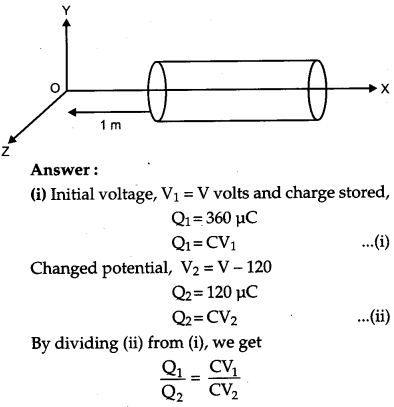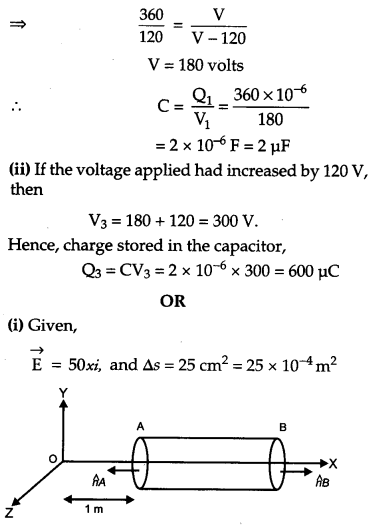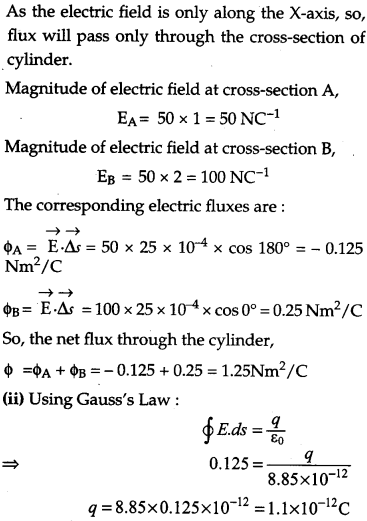Question 19.
(a) In a typical nuclear reaction, e.g.
$$_{1}^{2} \mathrm{H}+_{1}^{2} \mathrm{H} \rightarrow_{2}^{3} \mathrm{He}+\frac{1}{0} n+3.27 \mathrm{MeV}$$ although number of nucleons is conserved, yet energy is released. How ? Explain.
(b) Show that nuclear density in a given nucleus is independent of mass number A. 
(a) In a nuclear reaction, the aggregate of the
masses of the target nucleus $$\overset { 2 }{ 1 } H$$ and the bombarding particle may be greater or less than the aggregate of the masses of the product nucleus $$\overset { 3 }{ 2 } H$$ and the outgoing particle $$\overset { 1 }{ 0 } H$$ So from the law of conservation of mass-energy some energy (3.27 MeV) is evolved or involved in a nuclear reaction. This energy is called Q-value of the nuclear reaction.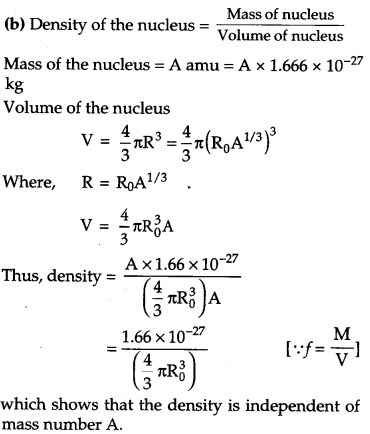Question 20.
(a) Why photoelectric effect cannot be explained on the basis of wave nature of light ? Give reasons.
(b) Write the basic features of photon picture of electro magnetic radiation on which Einstein’s photoelectric equation is based. 
(a) Wave nature of radiation cannot explain the following : .

1. The immediate ejection of photo electrons.
2. The presence of threshold frequency for a metal surface.
3. The fact that kinetic energy of the emitted electrons is independent of the intensity of light and depends upon its frequency. Thus, the photoelectric effect cannot be explained on the basis of wave nature of light.

(b) Photon picture of electromagnetic radiation on which Einstein’s photoelectric equation is based on particle nature of light. Its basic features are :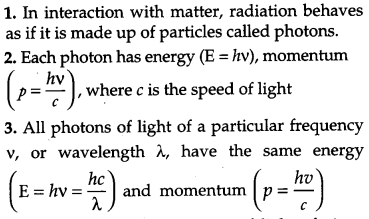4. By increasing the intensity of light of given wavelength, there is only an increase in the number of photons emitted per second crossing a given area, with each photon having the same energy. Thus, photon energy is independent of intensity of radiation.

5. Photons are electrically neutral and are not deflected by electric and magnetic fields.

6. In a photon-particle collision (such as photon- electron collision), the total energy and total momentum are conserved. However, number of photons may not be Observed.

Question 21.
A metallic rod of length ‘l’ is rotated with a frequency v with one end hinged at the center and the other end at the circumference of a circular metallic ring of radius r, about an axis passing through the center and perpendicular to the plane of the ring. A constant uniform magnetic field B parallel to the axis is present everywhere. Using Lorentz force, explain how emf is induced between the center and the metallic ring and hence obtained the expression for it. 
Suppose the length of the rod is greater than the radius of the circle and rod rotates anticlockwise and suppose the direction of electrons in the rod at any instant be along +y direction.
Suppose the direction of the magnetic field is along +z direction.
Then, using Lorentz law, we get the following :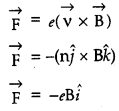Thus, the direction of force on the electrons is along -x axis. So, the electrons will move towards the centre i.e., the fixed end of the rod. This movement of electrons will effect in current and thus it will generate an emf in the rod between the fixed end and the point touching the ring.

Let θ be the angle between the rod and radius of the circle at any time t.
Then, area swept by the rod inside the circle =Question 22.
Output characteristics of an n-p-n transistor in CE configuration is shown in the figure. 
Determine:
(i) dynamic output resistance
(ii) dc current gain and
(iii) ac current gain at an operating point VCE = 10 V, when IB = 30 μA.Question 23.
Using Bohr’s postulates, obtain the expression for the total energy of the electron in the stationary states of the hydrogen atom. Hence draw the energy level diagram showing how the line spectra corresponding to Balmer series occur due to transition between energy levels. 
In a hydrogen atom, Radius of electron orbit,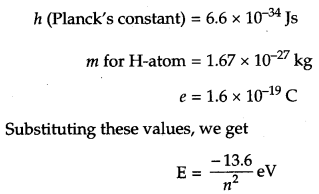When the electron in a hydrogen atom jumps from higher energy level to the lower energy level, the difference of energies of the two energy levels is emitted as a radiation of particular wavelength. It is called a spectral line.
In H-atom, when an electron jumps from the orbit ni, to orbit nf, the wavelength of the emitted radiation is given by,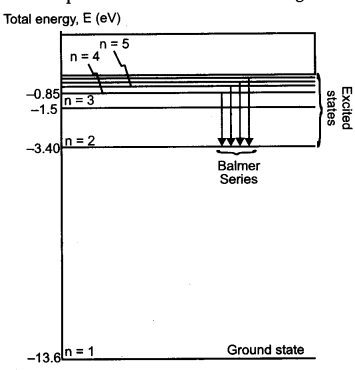Question 24.
(a) In what way is diffraction from each slit related to the interference pattern in a double slit experiment.
(b) Two wavelengths of sodium light 590 nm and 596 nm are used, in turn to study the diffraction taking place at a single slit of aperture 2 x 10-4 m. The distance between the slit and the screen is 1.5 m. Calculate the separation between the positions of the first maxima of the diffraction pattern obtained in the two cases. 
(a) If the width of each slit is comparable to the wavelength of light used, the interference pattern thus obtained in the double slit experiment is modified by diffraction from each of the two slits.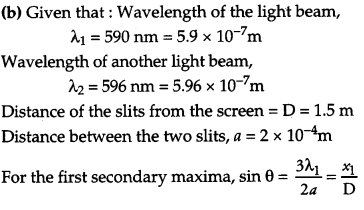Question 25.
In a series LCR circuit connected to an ac source of variable frequency and voltage v = vm sin ωt, draw a plot showing the variation of current (I) with angular frequency (ω) for two different values of resistance R1 and R2 (R1 > R2). Write the condition under which the phenomenon of resonance occurs. For which value of the resistance out of the two curves, a sharper resonance is produced ? Define Q-factor of the circuit and give its significance. 
Figure shows the variation of im with ω in a LCR series circuit for two values of Resistance R1 and R2 (R1 > R2),The condition for resonance in the LCR circuit is,
$$\omega_{0}=\frac{1}{\sqrt{L C}}$$

We can observe that the current amplitude is maximum at the resonant frequency (ω0. Since im = Vm/R at resonance, the current amplitude for case R2 is sharper to that for case R1.
Quality factor or simply the Q-factor of a resonant LCR circuit is defined as the ratio of voltage drop across the capacitor (or inductor) to that of applied voltage.
It is given by $$\mathrm{Q}=\frac{1}{\mathrm{R}} \sqrt{\frac{\mathrm{L}}{\mathrm{C}}}$$

The Q factor determines the sharpness of the resonance curve and if the resonance is less sharp the maximum current decreases and also the circuit is close to the resonance for a larger range Δω of frequencies and the regulation of the circuit will not be good. So, less sharp the resonance, less is the selectivity of the circuit while higher is the Q, sharper is the resonance curve and lesser will be the loss in energy of the circuit and circuit will be more selective.

Question 26.
While travelling back to his residence in the car, Dr. Pathak was caught up in a thunderstorm. It became very dark. He stopped driving the car and waited for thunderstorm to stop. Suddenly he noticed a child walking alone on the road. He asked the boy to come inside the car till the thunderstorm stopped. Dr. Pathak dropped the boy at his residence. The boy insisted that Dr. Pathak should meet his parents. The parents expressed their gratitude to Dr. Pathak for his concern for safety of the child.
Answer the following questions based on the above information: 
(a) Why is it safer to sit inside a car during a thunderstorm ?
(b) Which two values are displayed by Dr. Pathak in his action ?
(c) Which values are reflected in parents’ response to Dr. Pathak ?
(d) Give an example of similar action on your part in the part from everyday life.
(a) It is safer to be inside a car during thunderstorm because the car acts like a Faraday cage.
(d) Once I came across to a situation where a puppy was struck in the middle of a busy road during rain and was not able to cross due to heavy flow, So I quickly rushed and helped him.

Question 27.
(a) Draw a ray diagram showing the image formation by a compound microscope. Hence obtain expression for total magnification when the image is formed at infinity.
(b) Distinguish between myopia and hypermetropia. Show diagrammatically how these defects can be corrected. 
OR
(a) State Huygens’s principle. Using this principle draw a diagram to show how a plane wave front incident at the interface of the two media gets refracted when it propagates from a rarer to a denser medium. Hence verify Snell’s law of refraction.
(b) When monochromatic light travels from a rarer to a denser medium, explain the following, giving reasons:
(i) Is the frequency of reflected and refracted light same as the frequency of incident light ?
(ii) Does the decrease in speed imply a reduction in the energy carried by light wave ?
(a) A compound microscope consists of two convex lenses parallel separated by some distance. The lens nearer to the object is called the objective. The lens through which the final image is viewed is called the eyepiece.

infinity : The magnification produced by the compound microscope is the product of the magnifications produced by the eyepiece and objective.
∴ M = Me x M0

Where, Me and M0 are the magnifying powers of the eyepiece and objective respectively.
If μ0 is the distance of the object from the objective and v0 is the distance of the image from the objective, then the magnifying power of the objective is
$$\mathrm{M}_{o}=\frac{h^{\prime}}{h}=\frac{\mathrm{L}}{f_{o}}$$

Where, h, h’ are object and image heights respectively and f0 is the focal length of the objective and L is the tube length i.e., the distance between the second focal point of the objective and the first focal point of the eyepiece.
When the final image is at infinity,
$$\mathrm{M}_{e}=\frac{\mathrm{D}}{f_{e}}$$
Magnifying power of compound microscope,
$$\mathbf{M}=\mathbf{M}_{o} \times \mathbf{M}_{e}=\frac{\mathbf{L}}{f_{o}} \times \frac{\mathbf{D}}{f_{e}}$$
Magnifying power when final image is at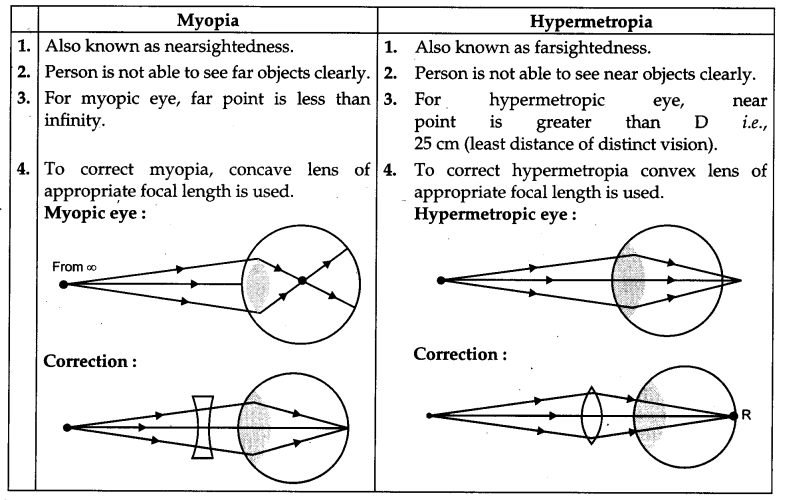OR
a) Huygen’s Principle:

1. Each point on the primary wave front acts as a source of secondary wavelets, transferring out disturbance in all directions in the same way as the original source of light does.
2. The new position of the wave front at any instant is the envelope of the secondary wavelets at that instant.

Refraction on the basis of wave theory:

1. Consider any point Q on the incident wave front.
2. Suppose when disturbance from point P on incident wave front reaches point P’ on the refracted wave front, the disturbance from point Q reaches Q’ on the refracting surface XY.3. Since P’A’ represents the refracted wave front, the time taken by light to travel from a point on incident wave front to the corresponding point on refracted wave front should always be the same.
Now, time taken by light to go from Q to Q’ will beThe rays from different points on the incident wave front will take the same time to reach the corresponding points on the refracted wave front i.e., given equation (iv) is independent of AK. It will happen so, if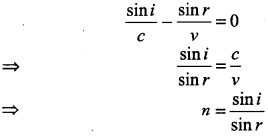This is the Snell’s law for refraction of light.
(b) (i) The frequency of reflected and refracted light remains constant as the frequency of incident light because frequency only depends on the source of light.
(ii) Since the frequency remains same, hence there is no reduction in energy.

Question 28.
(a) State the working principle of a potentiometer. With the help of the circuit diagram, explain how a potentiometer is used to compare the emf’s of two primary cells. Obtain the required expression used for comparing the emfs.
(b) Write two possible causes for one sided deflection in a potentiometer experiment. 
OR
(a) State Kirchhoff’s rules for an electric network. Using Kirchhoff’s rules, obtain the balance condition in terms of the resistance of four arms of Wheatstone bridge.
(b) In the meter bridge experimental set-up, shown in the figure, the null point ‘D’ is obtained at a distance of 40 cm from end A of the meter bridge wire. If a resistance of 10 Ω is connected in series with R1, null point is obtained at AD = 60 cm. Calculate the values of R1 and R2.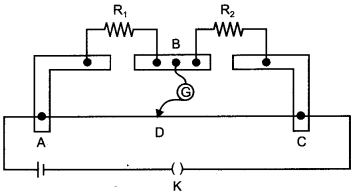(a) Working principle of Potentiometer : When a constant current is passed through a wire of uniform area of cross-section, the potential drop across any portion of the wire is directly proportional to the length of that portion.
Applications of Potentiometer for comparing emf’s of two cells : The following figure shows an application of the potentiometer to compare the emf of two cells of emf E1 and E2.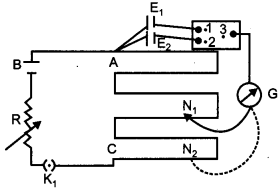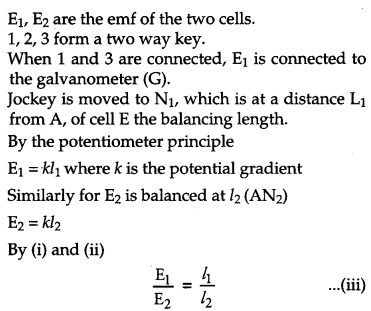we can compare the emf’s of any two sources. Generally, one of the cells is chosen as a standard cell whose emf is known to a high degree of accuracy. The emf of the other cell is then calculated from equation (iii).
(b) (i) The emf of the cell connected in main circuit may not be more than the emf of the primary cells whose emfs are to be compared.
(ii) The positive ends of a cells are not connected to the same end of the wire.
OR
(a) Kirchhoff’s first law – Junction rule : The algebraic Siam of the currents meeting at a point in an electrical circuit is always zero.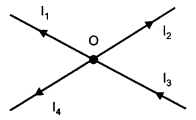Let the currents be Ii, I2,13, and I4.
Convention : Current towards the junction positive
Current away from the junction negative
I3 + (-I1) + (-I2) + (-14) = 0
This law is in accordance with conservation of charge

Kirchhoff’s second law-Loop rule : In a closed loop, the algebraic sum of the emfs is equal to the algebraic sum of the products of the resistance and current flowing through themWheatstone bridge : The Wheatstone bridge is an arrangement of four resistances as shown in the following figure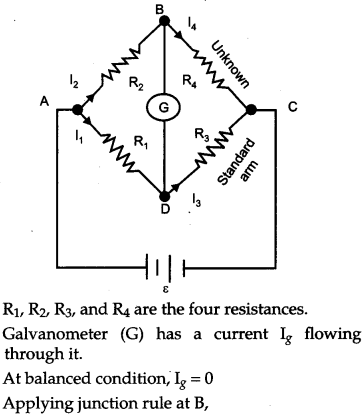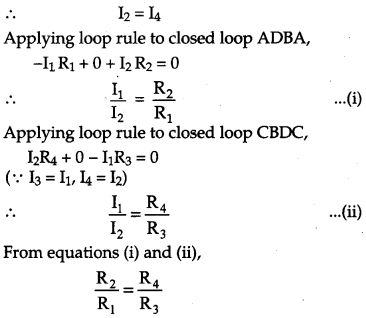This is the required balanced condition of Wheatstone bridge.
(b) Considering both the situations and writing them in the form of equations Let R’ be the resistance per unit length of the potentiometer wire,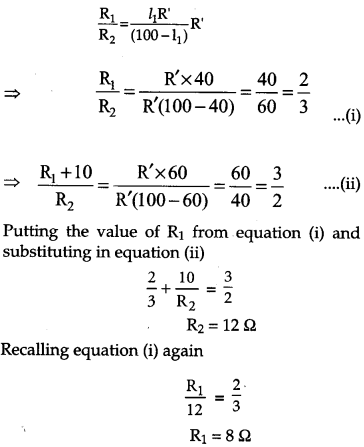Question 29.
(a) Derive the expression for the torque on a rectangular current carrying loop suspended in a uniform magnetic field.
(b)A proton and a deuteron having equal momenta enter in a region of a uniform magnetic field at right angle to the direction of the field. Depict their trajectories in the field. 
OR
(a) A small compass needle of magnetic moment’m’ is free to turn about an axis perpendicular to the direction of uniform magnetic field ‘B’. The moment of inertia of the needle about the axis is ‘I’. The needle is slightly disturbed from its stable position and then released. Prove that it executes simple harmonic motion. Hence deduce the expression for its time period.
(b) A compass needle, free to turn in a vertical plane orients itself with its axis vertical at a certain place on the earth. Find out the values of
(i) horizontal component of earth’s magnetic field and
(ii) angle of dip at the place.
(a) Consider a rectangular loop PQRS of length l, breadth b suspended in a uniform magnetic field $$\overrightarrow{\mathrm{B}}$$ . The length of loop PQ = RS = l and breadth = QR = SP = b. Let at any instant the normal to the plane of loop make an angle θ with the direction of magnetic field $$\overrightarrow{\mathrm{B}}$$ and I be the current in the loop. We know that a force acts on a current carrying wire placed in a magnetic field. Therefore, each side of the loop will experience a force. The net torque acting on the loop will be determined by the forces acting on all sides of the loop. Suppose that the forces on sides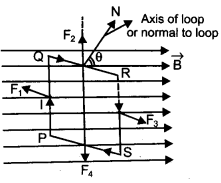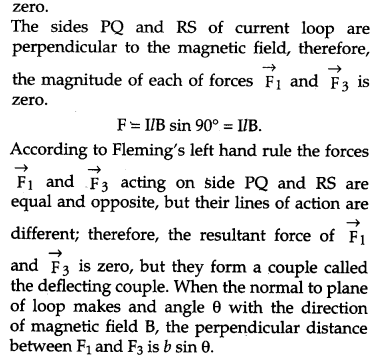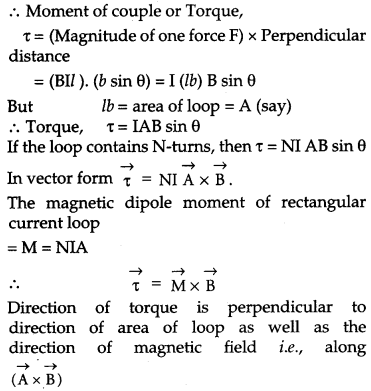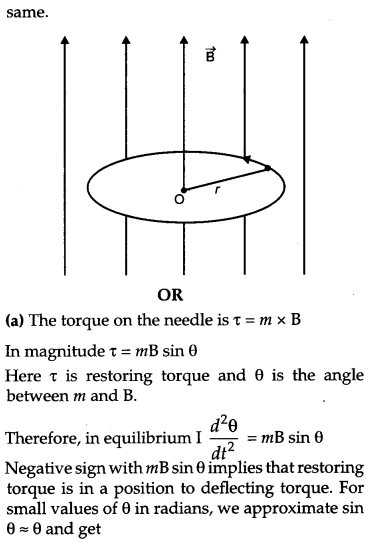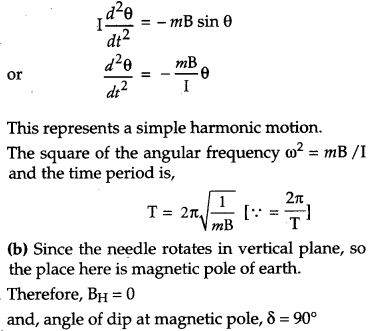### CBSE Previous Year Question Papers Class 12 Physics 2013 Delhi Set-II

Note: Except for the following questions, all the remaining questions have been asked in previous Set.

Question 1.
A cell of emf ‘E’ and internal distance ‘r’ draws a current ‘I’. Write the relation between terminal voltage ‘V’ in terms of E, I, r. 
When the current I draws from a cell of emf E and internal resistance r, then the terminal voltage is
V = E – Ir

Question 2.
Which of the following substances are diamagnetic ? 
Bi, Al, Na, Cu, Ca and Ni
Bi and Cu are diamagnetic substances

Question 3.
A heating element is marked 210 V, 630 W. What is the value of current drawn by the element when connected to a 210 V dc source ? 
In dc source, P = VI
Given that P = 630 W and V = 210 V.
So,
$$I=\frac{P}{V}=\frac{630}{210}=3 \mathrm{A}$$

Question 4.
An ammeter of resistance 1Ω can measure current up to 1.0 A.
(i) What must be the value of the shunt resistance to enable the ammeter to measure upto 5.0 A ?
(ii) What is the combination resistance of the ammeter and the shunt ? 
We have, Resistance of ammeter,
RA = 1Ω
Maximum current across ammeter, IA = 1.0 A
So, Voltage drop across ammeter, V = IR = 1.0 × 1.0 = 1VQuestion 5.
A convex lens of focal length 20 cm is placed coaxially in contact with a concave lens of focal length 25 cm. Determine the power of the combination. Will the system be converging or diverging in nature ? 
We have, Focal length of concave lens f1 = +20 cm = +0.20 m,
Focal length of concave lens, f2 = -25 cm = -0.25 m
Power of convex lens,Question 6.
Using Bohr’s postulates, obtain the expressions for
(i) kinetic energy and
(ii) potential energy of the electron in stationary state of hydrogen atom.
Draw the energy level diagram showing how the transitions between energy levels result in the appearance of Lyman series. 
According to Bohr’s postulates, in a hydrogen atom, a single electron revolves around a nucleus of charge + e.
And the radius of orbit in which the electron revolves, is given byQuestion 7.
Figure shows a rectangular loop conducting PQRS in which the arm PQ is free to move. A uniform magnetic field acts in the direction perpendicular to the plane of the loop. Arm PQ is moved with a velocity v towards the arm RS Assuming that the arms QR, RS and SP have negligible resistances and the moving arm PQ has the resistance r, obtain the expression for
(i) the current in the loop
(ii) the force and
(iii) the power required to move the arm PQ.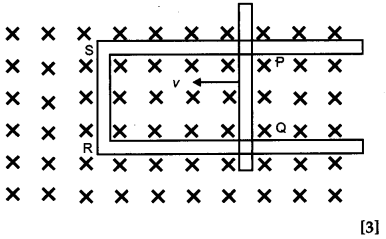Question 8.
Distinguish between ‘sky waves’ and ‘space waves’ modes of propagation in communication system. 
(a) Why is sky wave mode propagation restricted to frequencies upto 40 MHz ?
(b) Give two examples where space wave mode of propagation is used

### CBSE Previous Year Question Papers Class 12 Physics 2013 Delhi Set-III

Note : Except for the following questions, all the remaining questions have been asked in previous Sets.

Question 1.
A 5 V battery of negligible internal resistance is connected across a 200 V battery and a resistance of 39 Q as shown in the figure. Find the value of the current.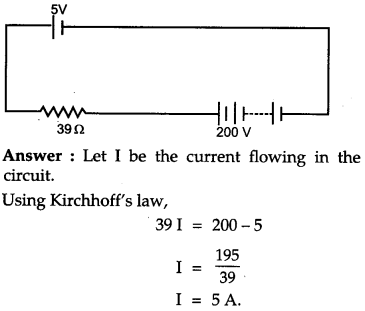Question 2.
Which of the following substances are paramagnetic ? 
Bi, Al, Cu, Ca, Pb, Ni
Paramagnetic substances are Aluminium (Al) and Calcium (Ca).

Question 3.
An ammeter of resistance 0.6 Ω can measure current up to 1.0 A. Calculate
(i) The shunt resistance required to enable the ammeter to measure current up to 5.0 A.
(ii) The combined resistance of the ammeter and the shunt. 
We have, resistance of ammeter, RA = 0.60 ohm.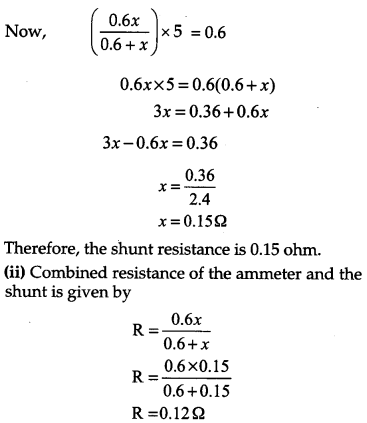Question 4.
A convex lens of focal length 30 cm is placed coaxially in contact with a concave lens of focal length 40 cm. Determine the power of the combination. Will the system be converging or diverging in nature ? 
We have, focal length of convex lens,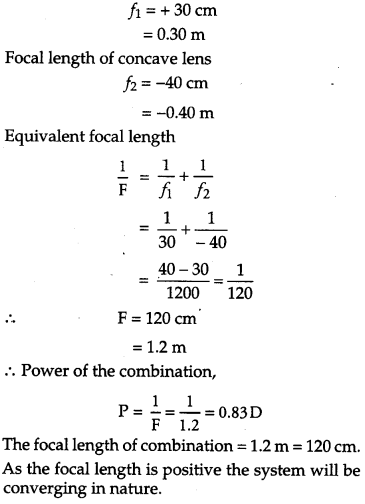Question 5.
A capacitor of unknown capacitance is connected across a battery of V volts. The charge stored in it is 300 μC. When potential across the capacitor is reduced by 100 V, the charge stored in it becomes 100 μC. Calculate the potential V and the unknown capacitance. What will be the charge stored in the capacitor if the voltage applied had increased by 100 V ? 
OR
A hollow cylindrical box of length 0.5 m and area of cross-section 20 cm is placed in a three-dimensional coordinate system as shown in the figure. The electric field in the region is given by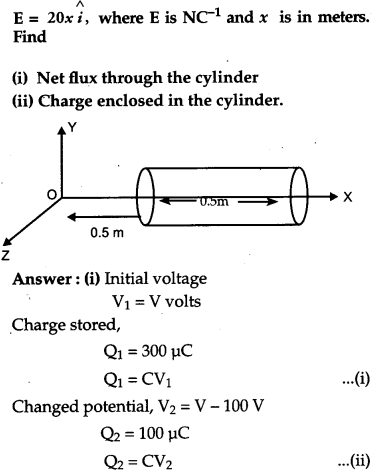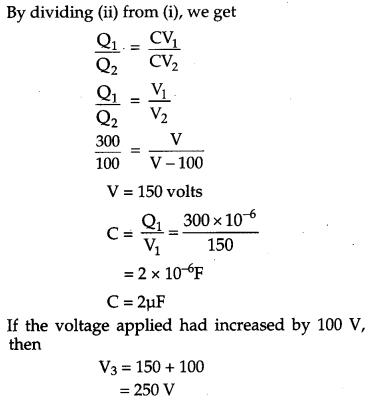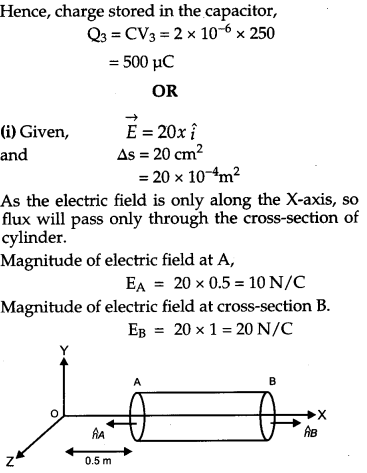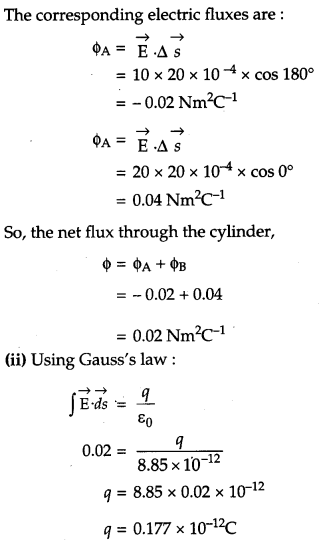Question 6.
(a) Write two characteristic features distinguishing the diffraction pattern from the interference fringes obtained in Young’s double slit experiment.
(b) Two wavelength of sodium light 590 nm and 596 nm are used, in turn, to study the diffraction taking place due to a single slit of aperture 1 × 10-4 m. The distance between the slit and the screen is 1.8 m. Calculate the separation between the positions of the first maxima of the diffraction pattern obtained in the two cases. 
(a) Two characteristic features distinguishing the diffraction pattern from the interference fringes obtained in Young’s double slit experiment are:

• The interference fringes may or may not be of the same width whereas the fringes of diffraction pattern are always of varying width.
• In interference the bright fringes are of same intensity whereas in diffraction pattern the intensity falls as we go to successive maxima away from the centre, on either side.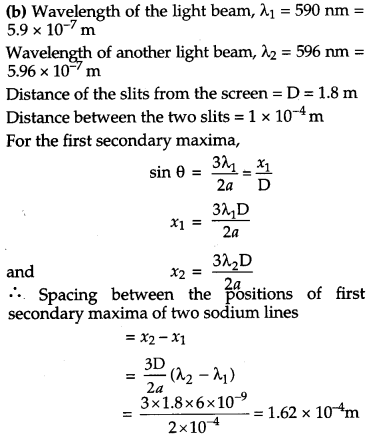Question 7.
(a) In a nuclear reaction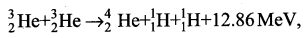though the number of nucleons is conserved on both sides of the reaction, yet the energy is released. How ? Explain.
(b) Draw a plot of potential energy between a pair of nucleons as a function of their separation. Mark the regions where potential energy is
(i) positive and
(ii) negative. 
(a) In a nuclear reaction, the sum of the masses of the target nucleus $$\overset { 2 }{ 3 } He$$ may be greater or less the sum of the masses of the product nucleus $$\overset { 4 }{ 2 } He$$ and the $$\overset { 1 }{ 1 } He$$ . So from the law of conservation of mass energy, some energy, (12.86 MeV) is evolved in nuclear reaction. This energy is called Q-value of the nuclear reaction. The binding energy of the nucleus on the left side is not equal to the right side. The difference in the binding energies on two sides appears as energy released or absorbed in the nuclear reaction.